ROOT   Reference Guidedouble32.C File Reference

## Detailed DescriptionTutorial illustrating use and precision of the Double32_t data type You should run this tutorial with ACLIC: a dictionary will be automatically created.

root > .x double32.C+

The following cases are supported for streaming a Double32_t type depending on the range declaration in the comment field of the data member:

Case Declaration
A Double32_t fNormal;
B Double32_t fTemperature; //[0,100]
C Double32_t fCharge; //[-1,1,2]
D Double32_t fVertex; //[-30,30,10]
E Double32_t fChi2; //[0,0,6]
F Int_t fNsp;
Double32_t* fPointValue; //[fNsp][0,3]
• Case A fNormal is converted from a Double_t to a Float_t
• Case B fTemperature is converted to a 32 bit unsigned integer
• Case C fCharge is converted to a 2 bits unsigned integer
• Case D the array elements of fVertex are converted to an unsigned 10 bits integer
• Case E fChi2 is converted to a Float_t with truncated precision at 6 bits
• Case F the fNsp elements of array fPointvalue are converted to an unsigned 32 bit integer. Note that the range specifier must follow the dimension specifier.

Case B has more precision than case A: 9 to 10 significative digits and 6 to 7 digits respectively. The range specifier has the general format: [xmin,xmax] or [xmin,xmax,nbits]. Examples

• [0,1]
• [-10,100];
• [-pi,pi], [-pi/2,pi/4],[-2pi,2*pi]
• [-10,100,16]
• [0,0,8] Note that:
• If nbits is not specified, or nbits <2 or nbits>32 it is set to 32
• If (xmin==0 and xmax==0 and nbits <=14) the double word will be converted to a float and its mantissa truncated to nbits significative bits.

## IMPORTANT NOTE

Lets assume an original variable double x. When using the format [0,0,8] (i.e. range not specified) you get the best relative precision when storing and reading back the truncated x, say xt. The variance of (x-xt)/x will be better than when specifying a range for the same number of bits. However the precision relative to the range (x-xt)/(xmax-xmin) will be worse, and vice-versa. The format [0,0,8] is also interesting when the range of x is infinite or unknown.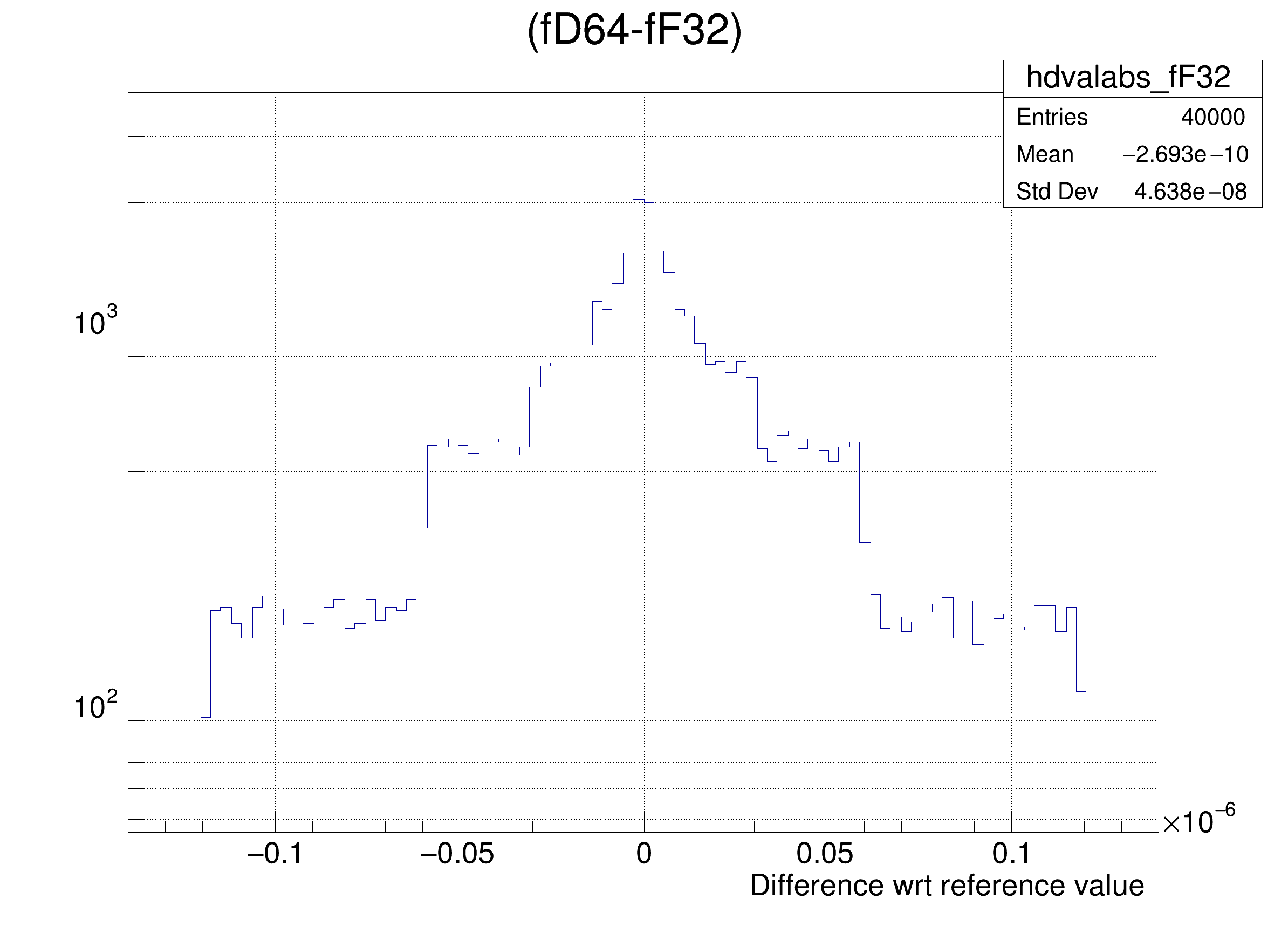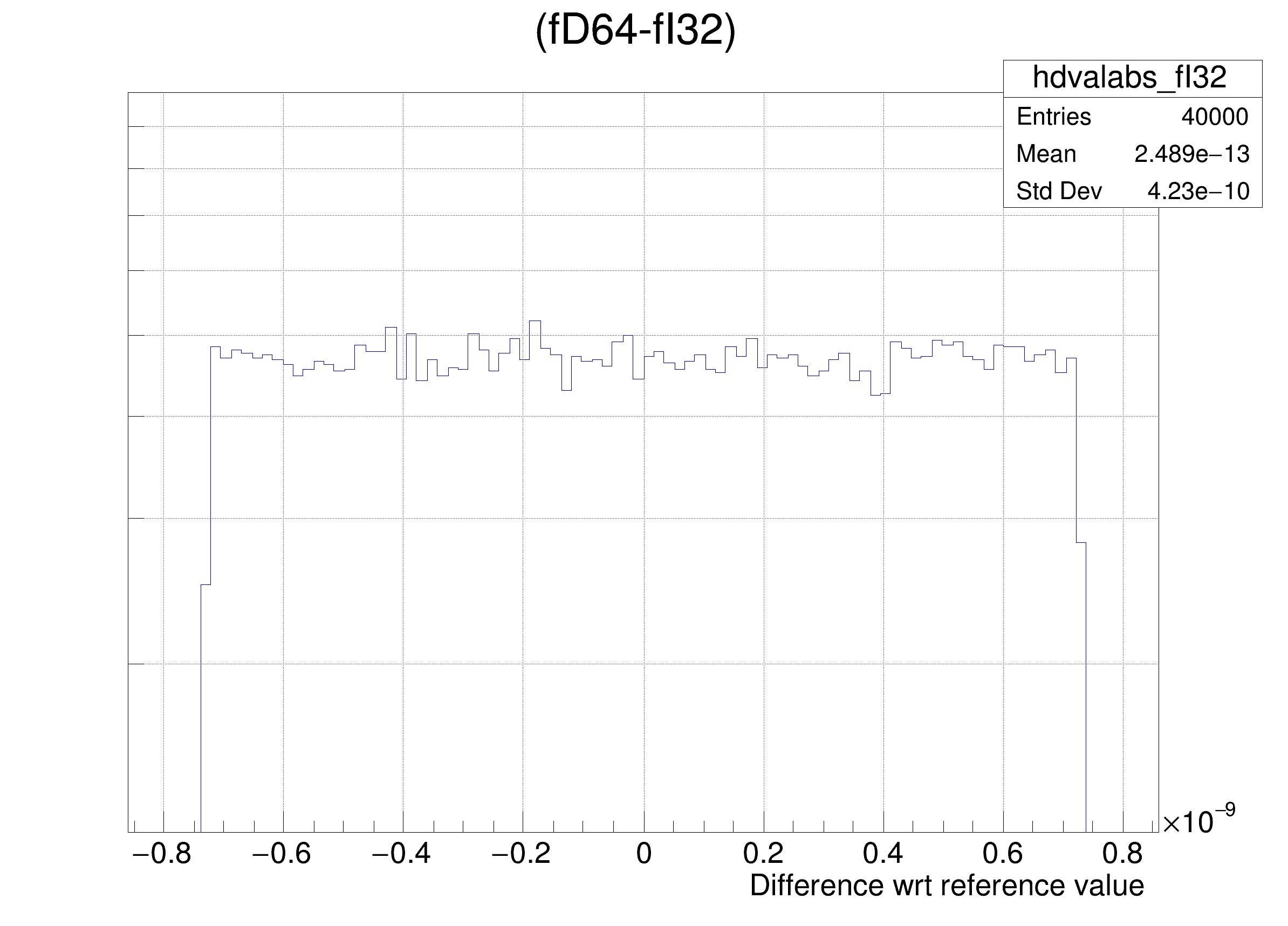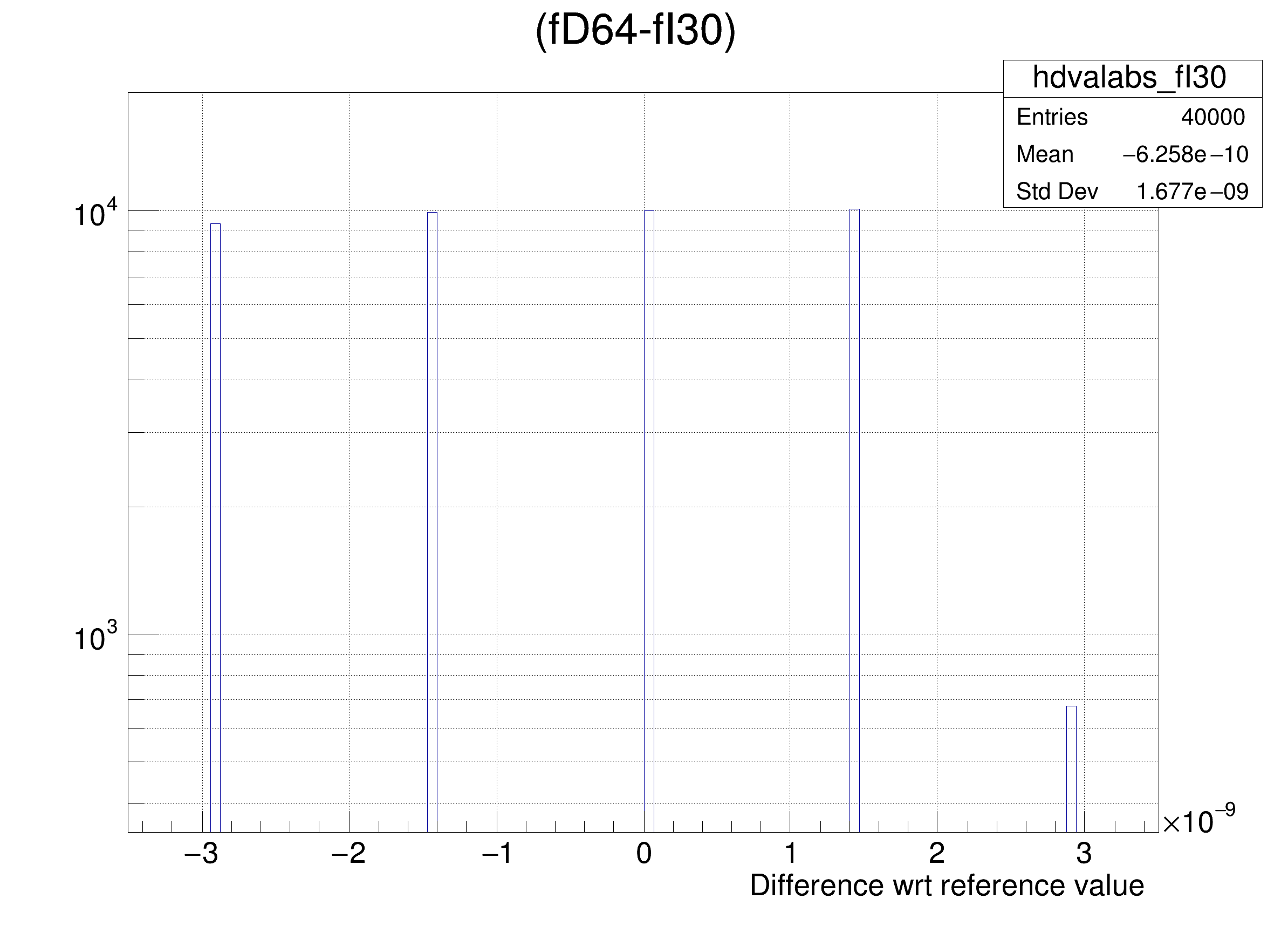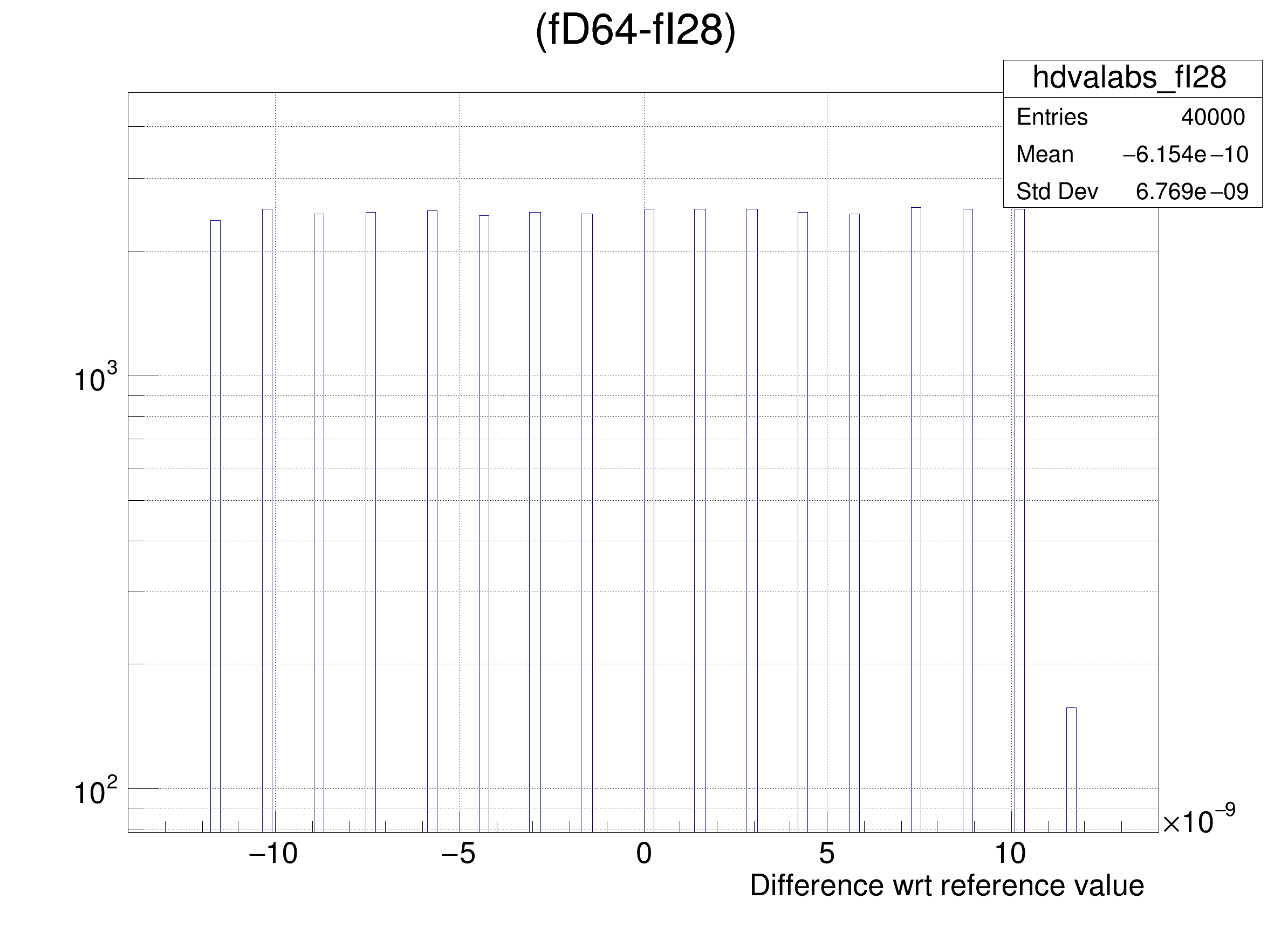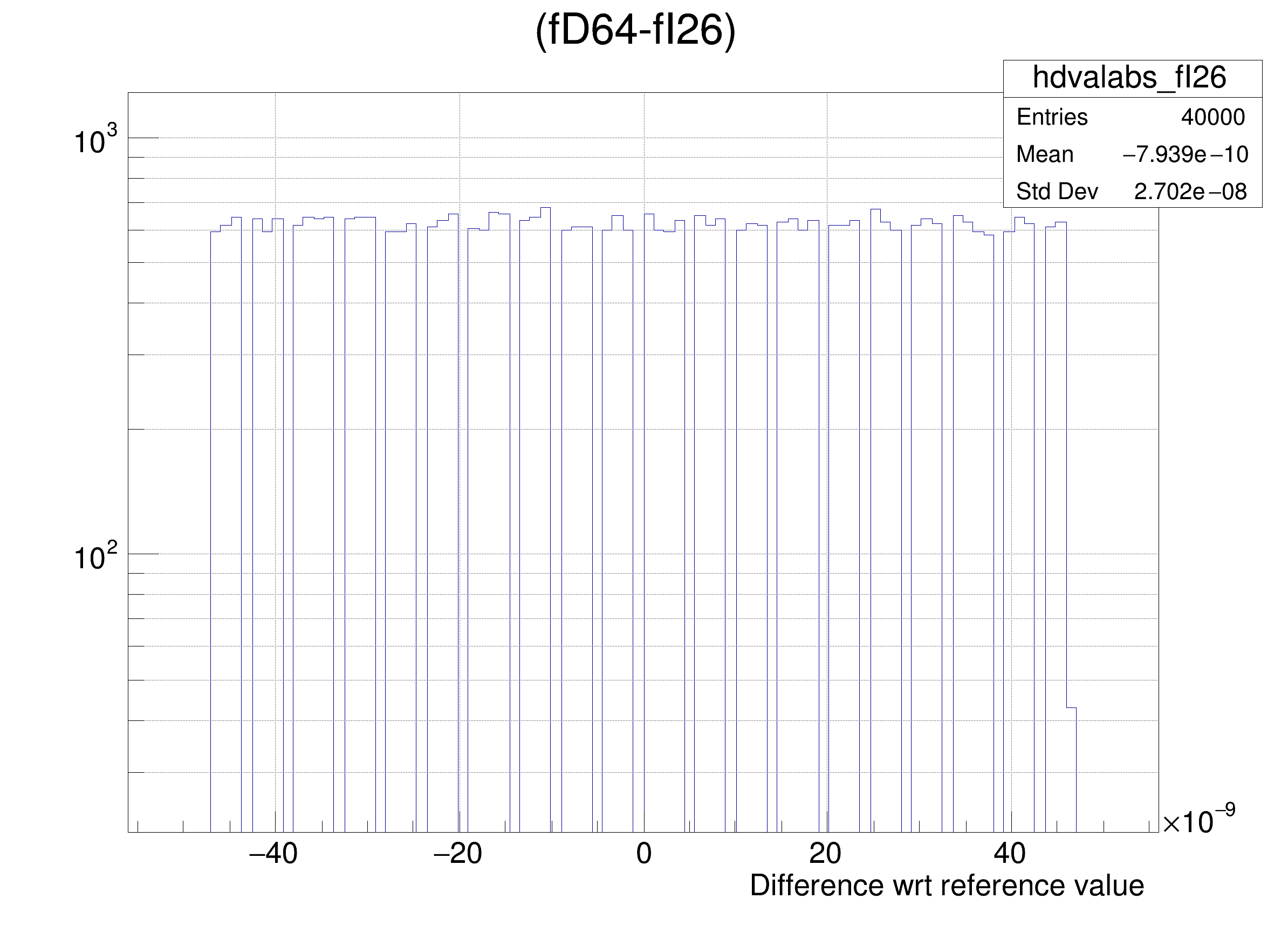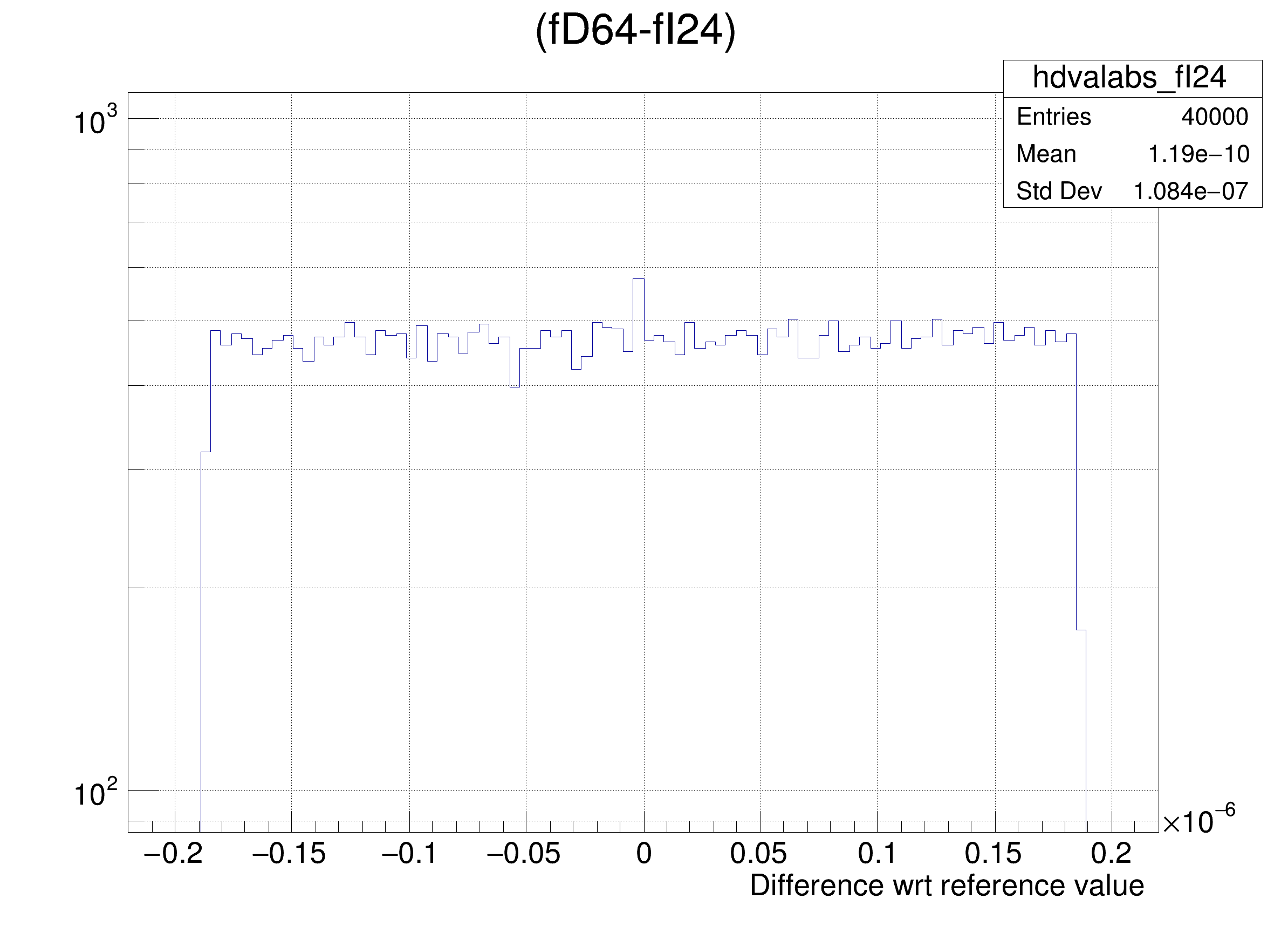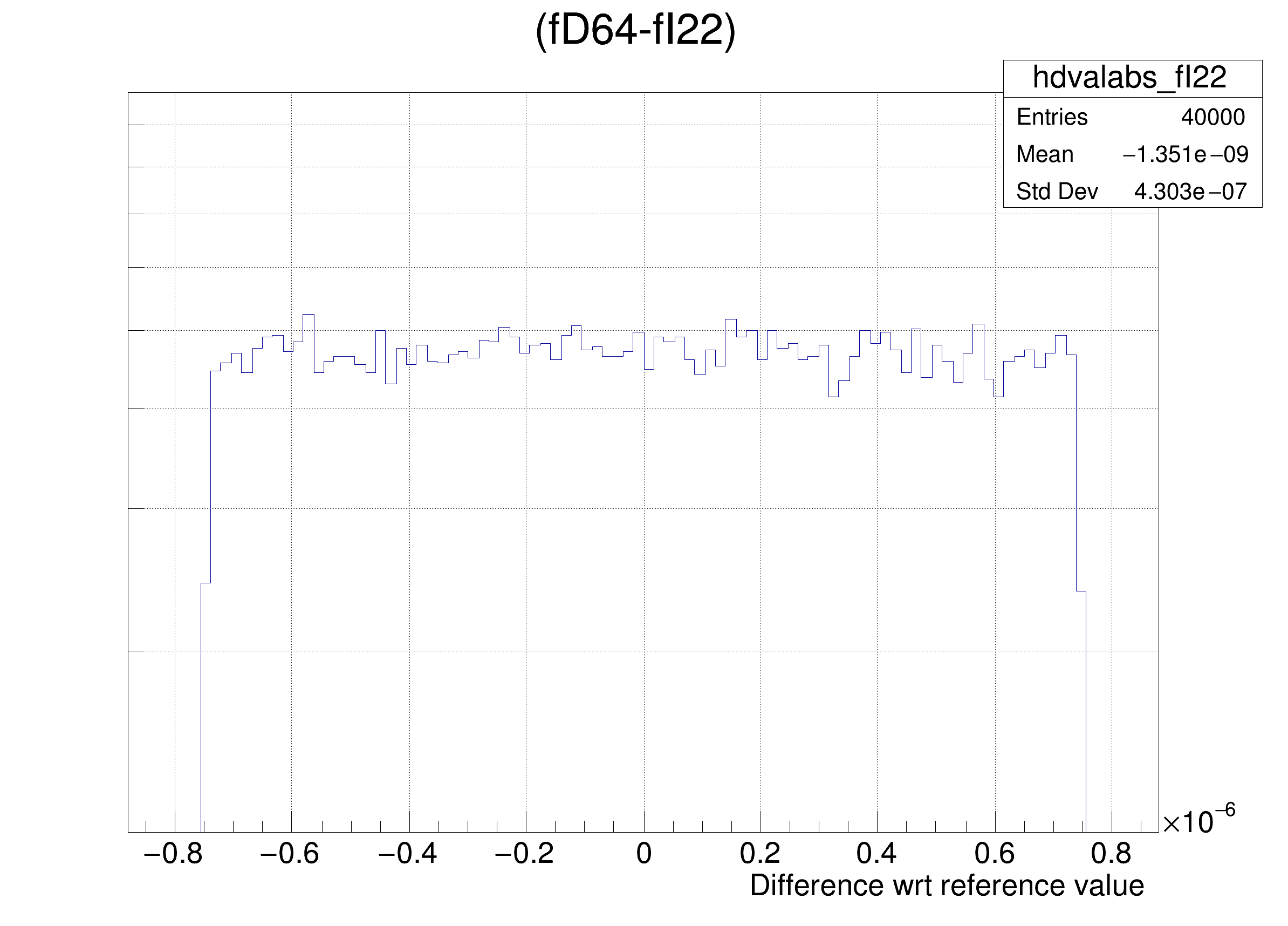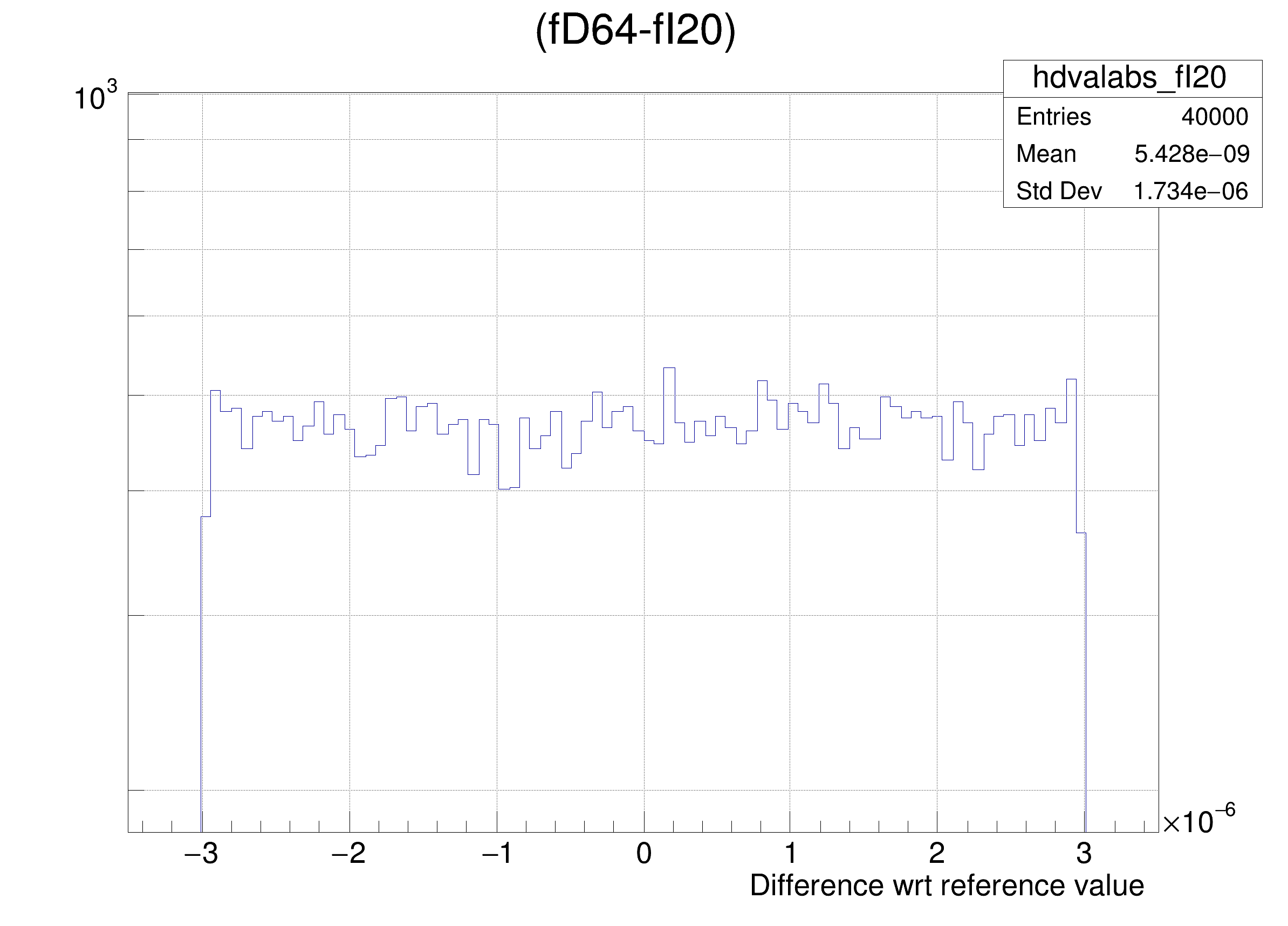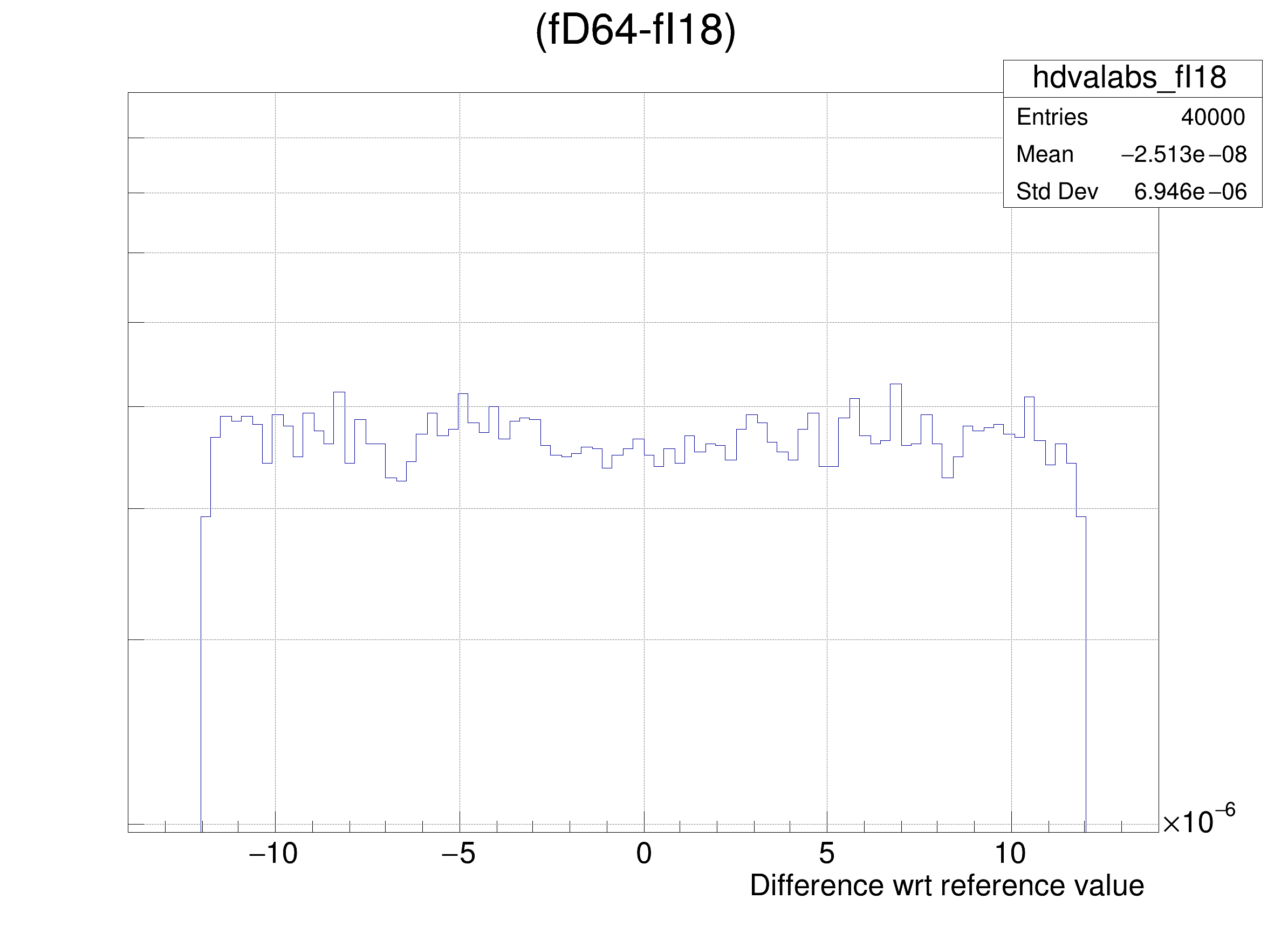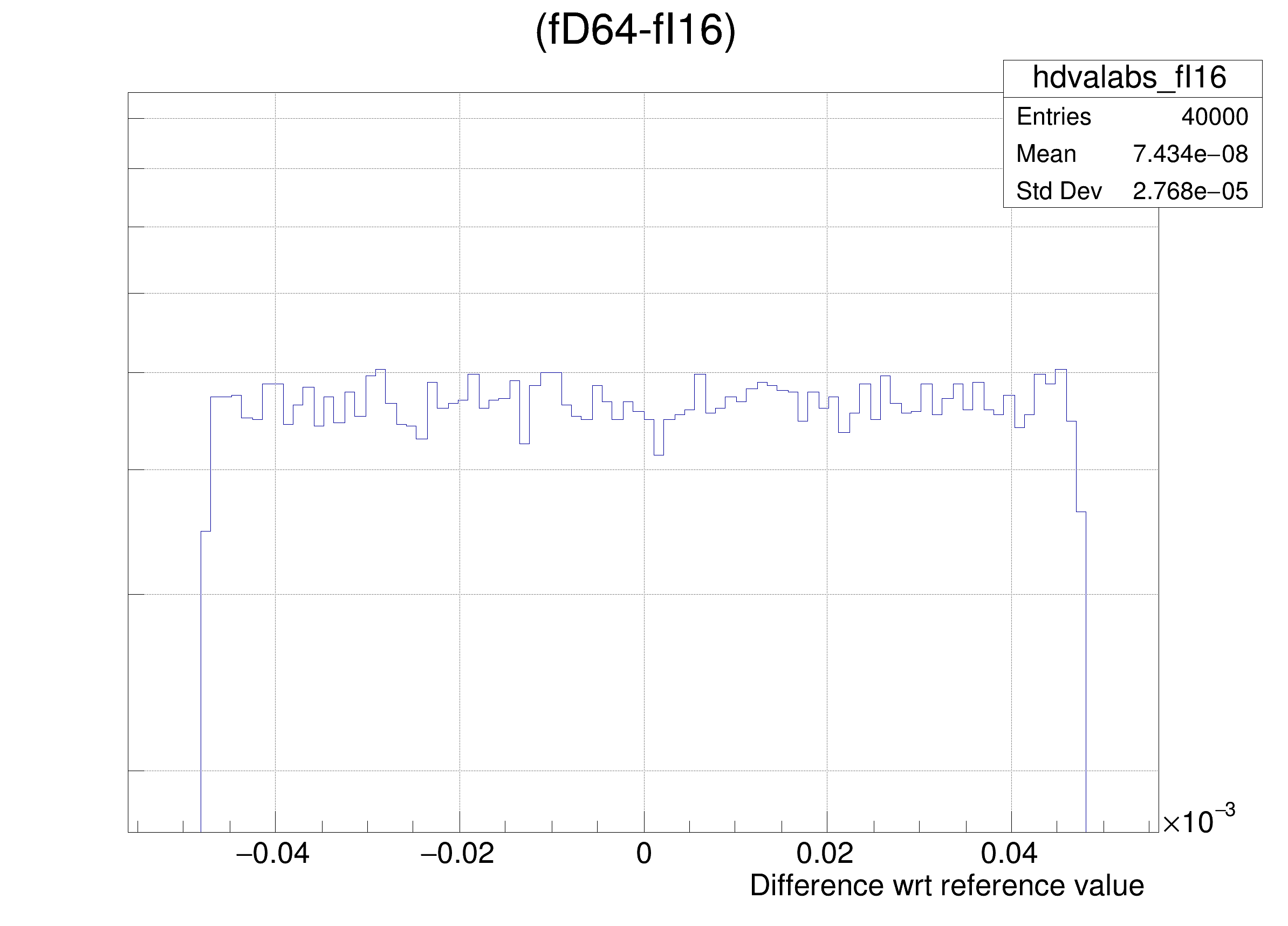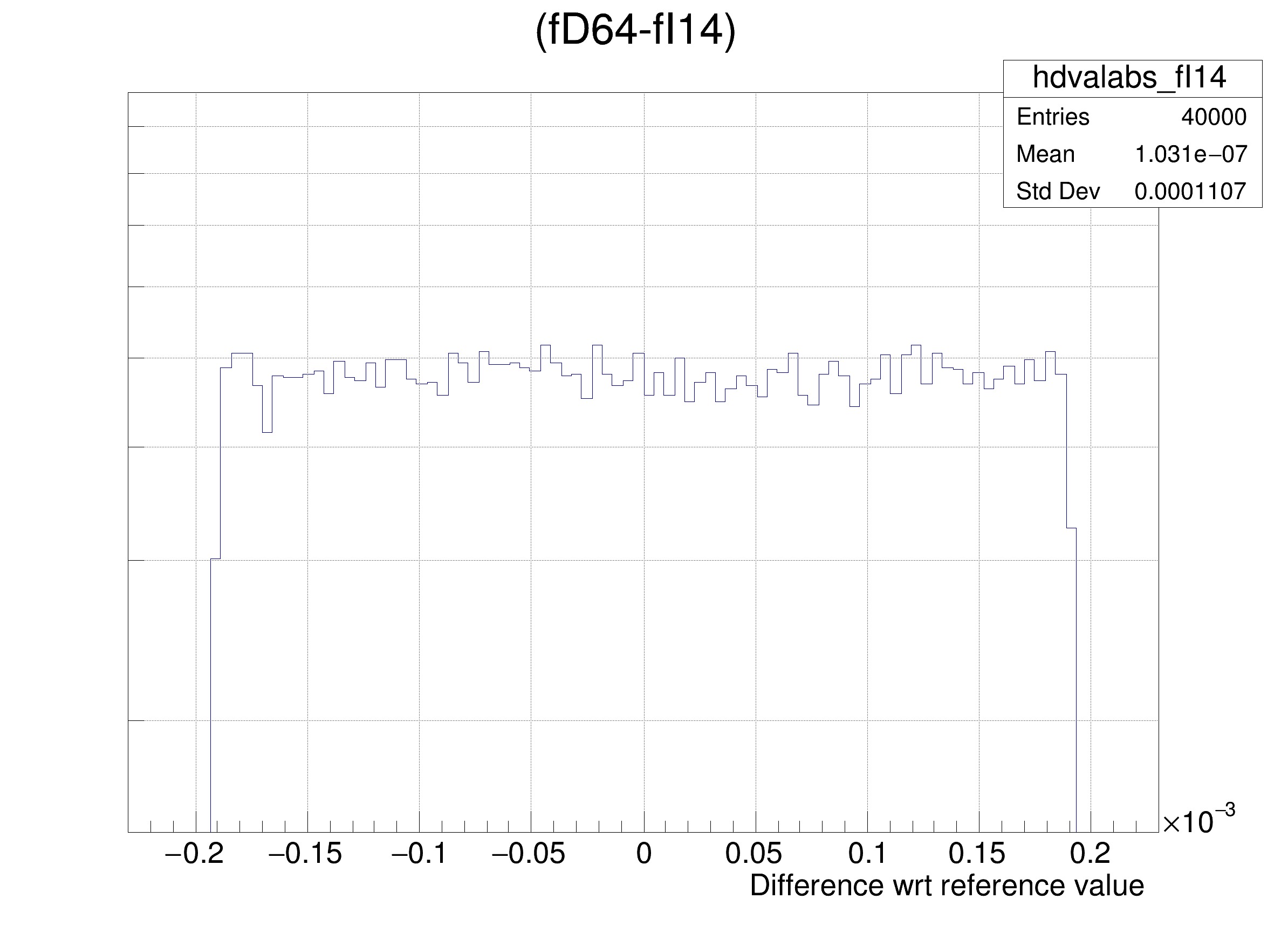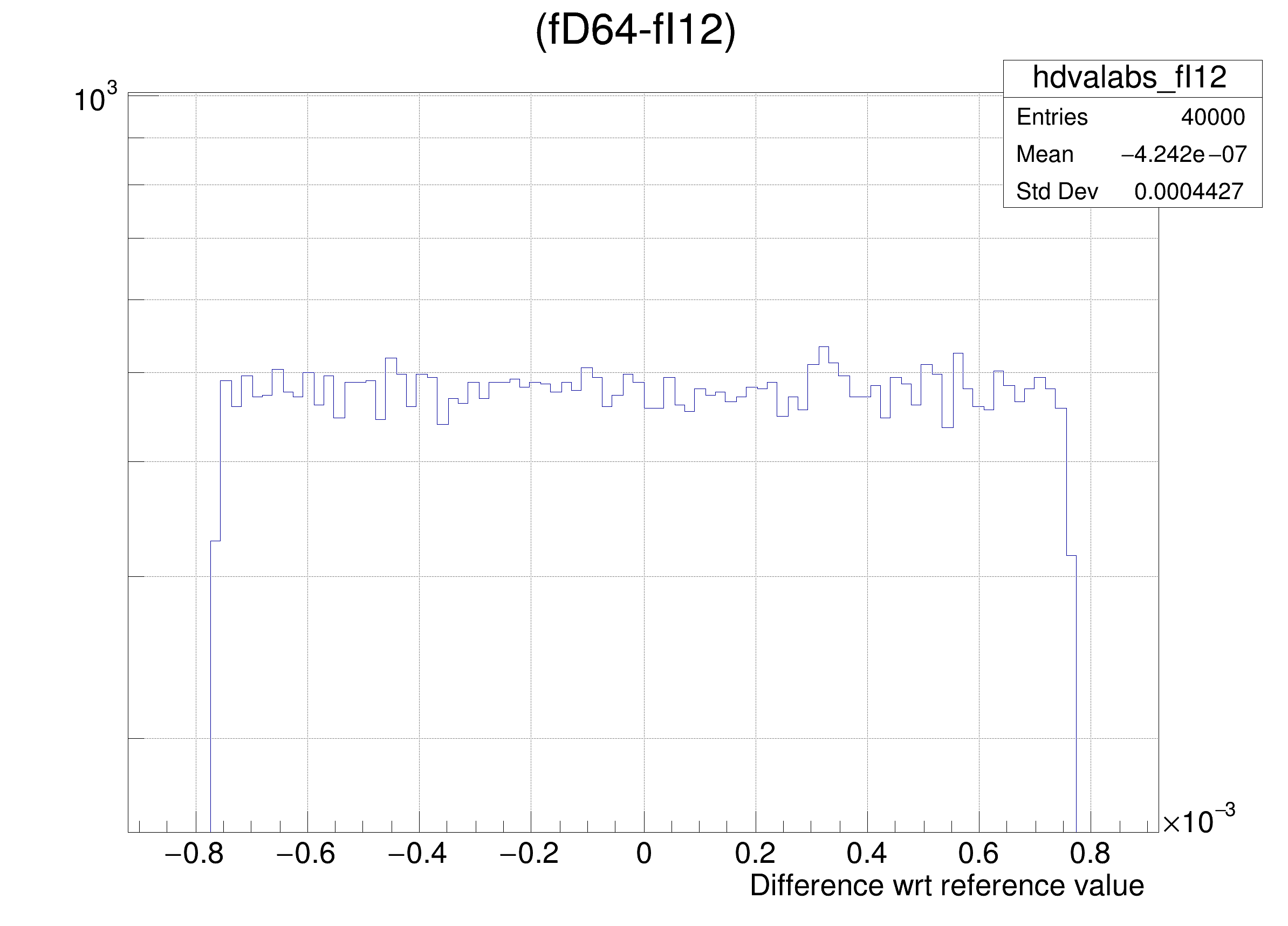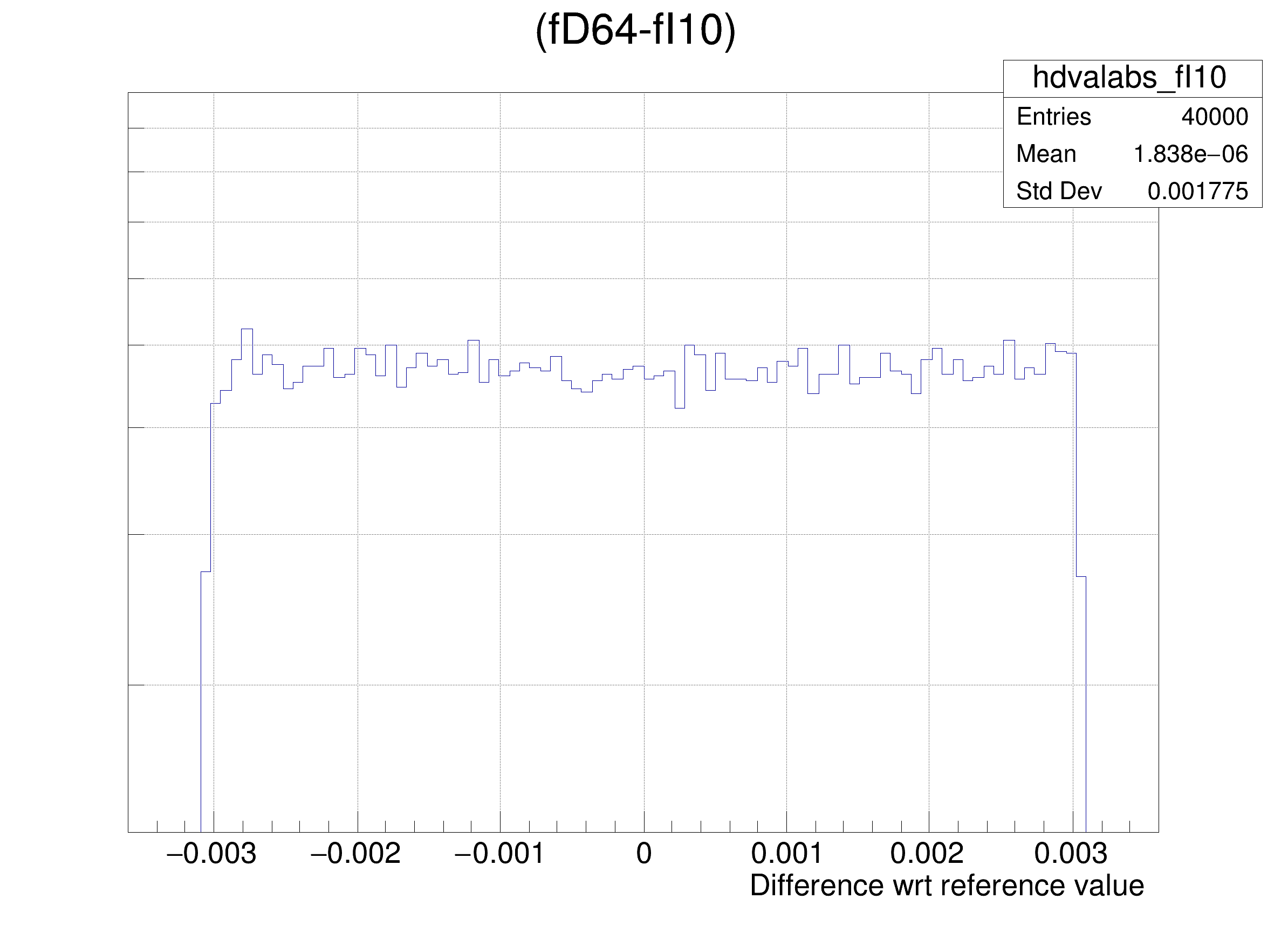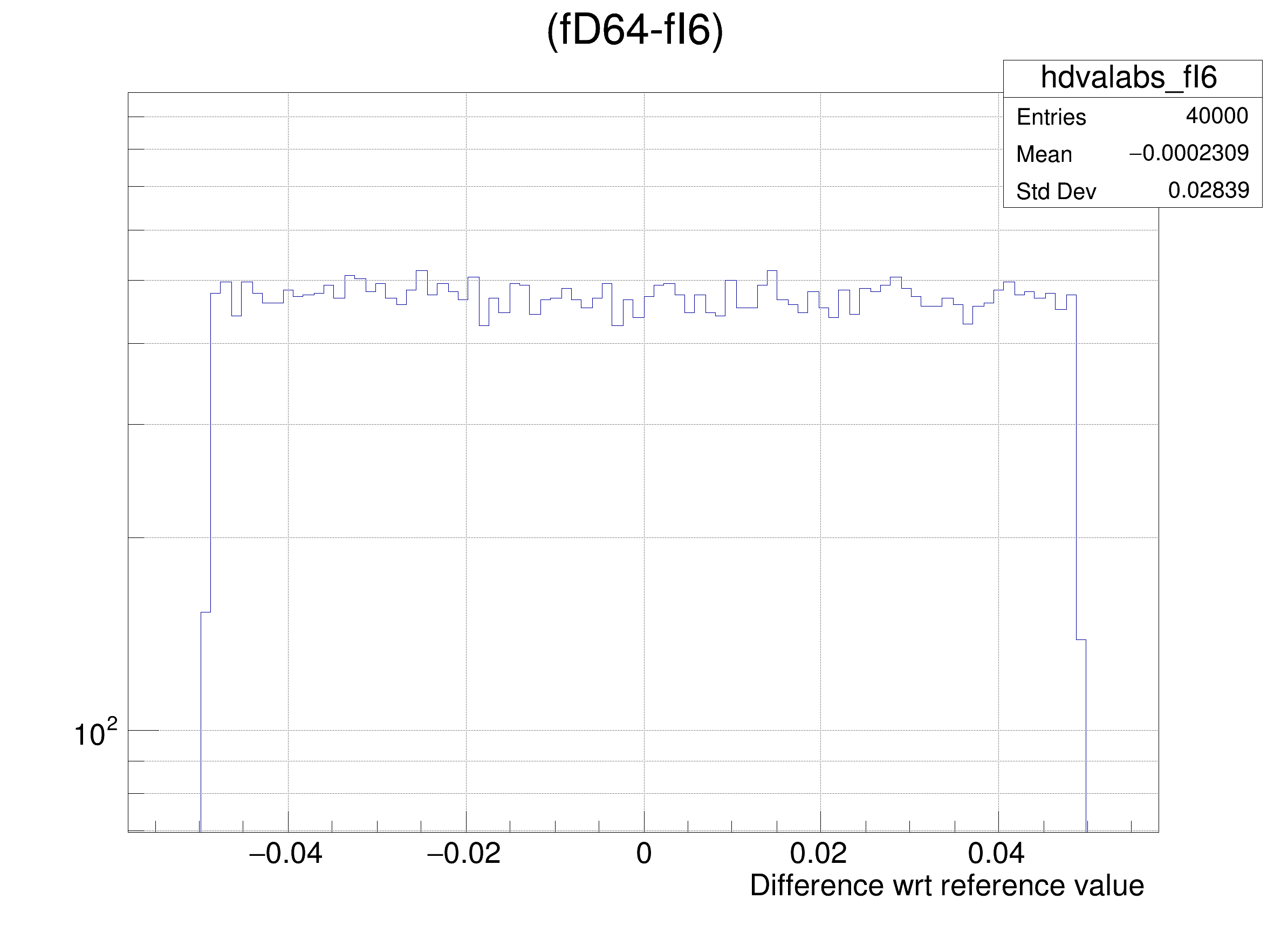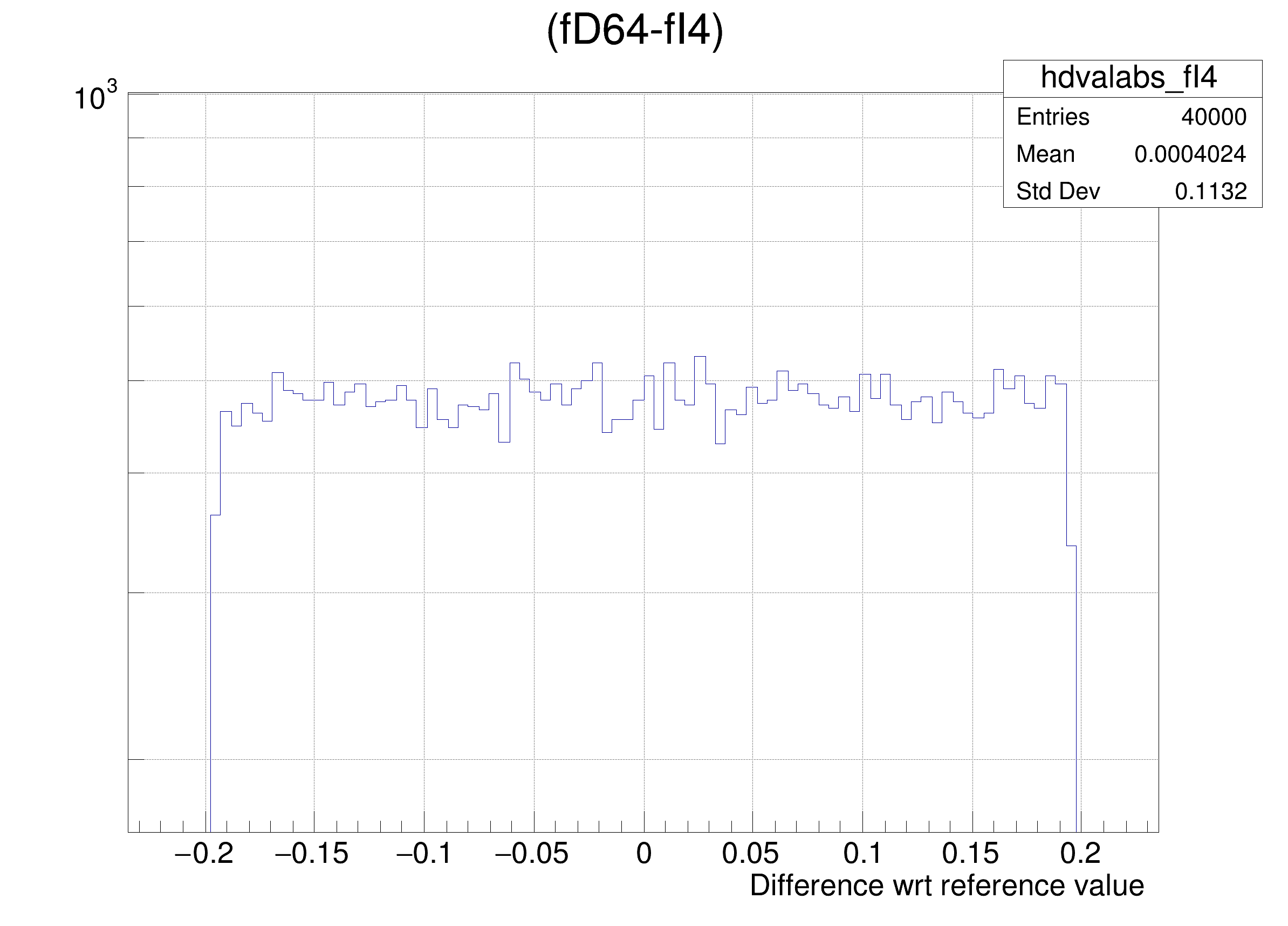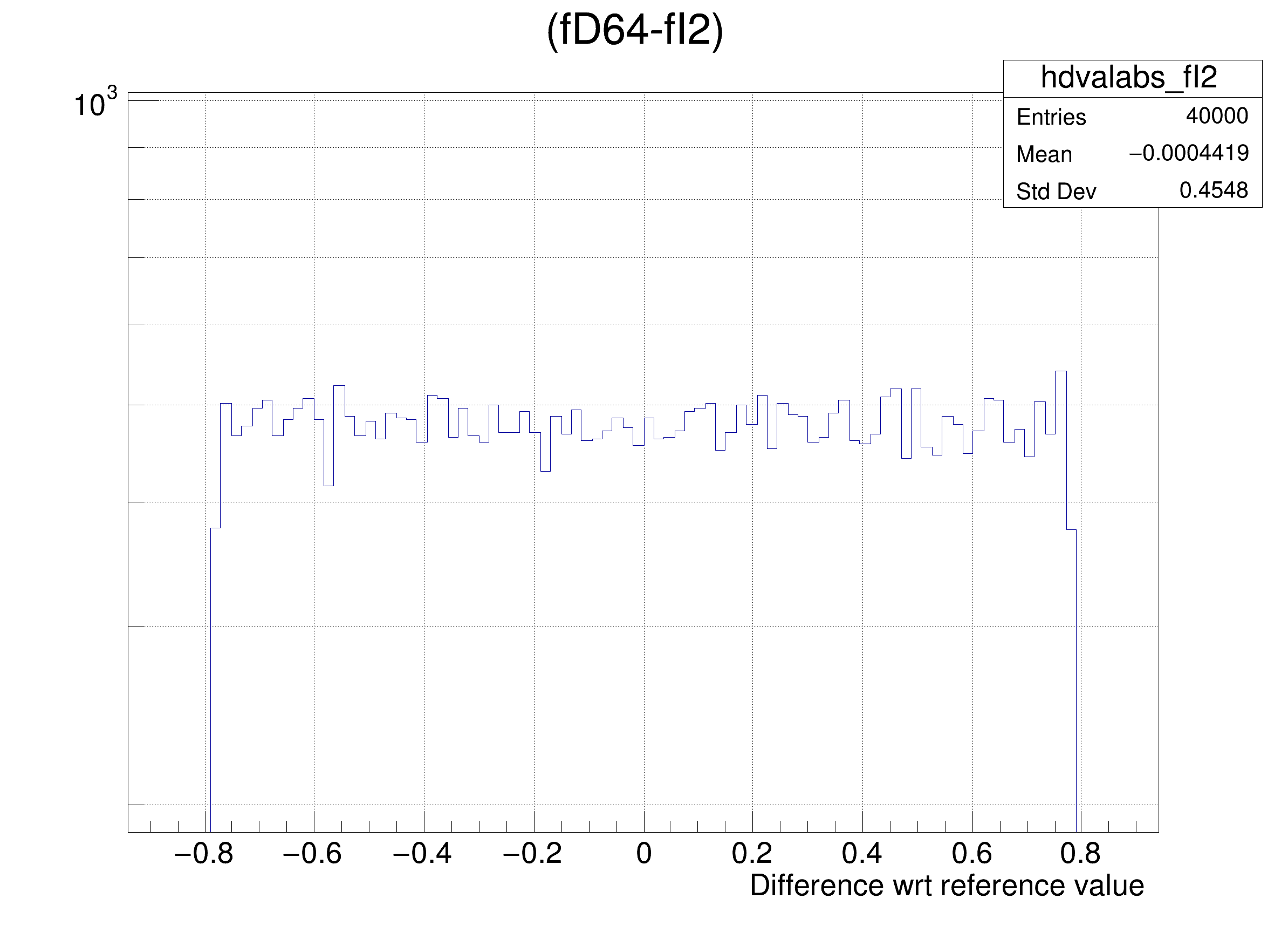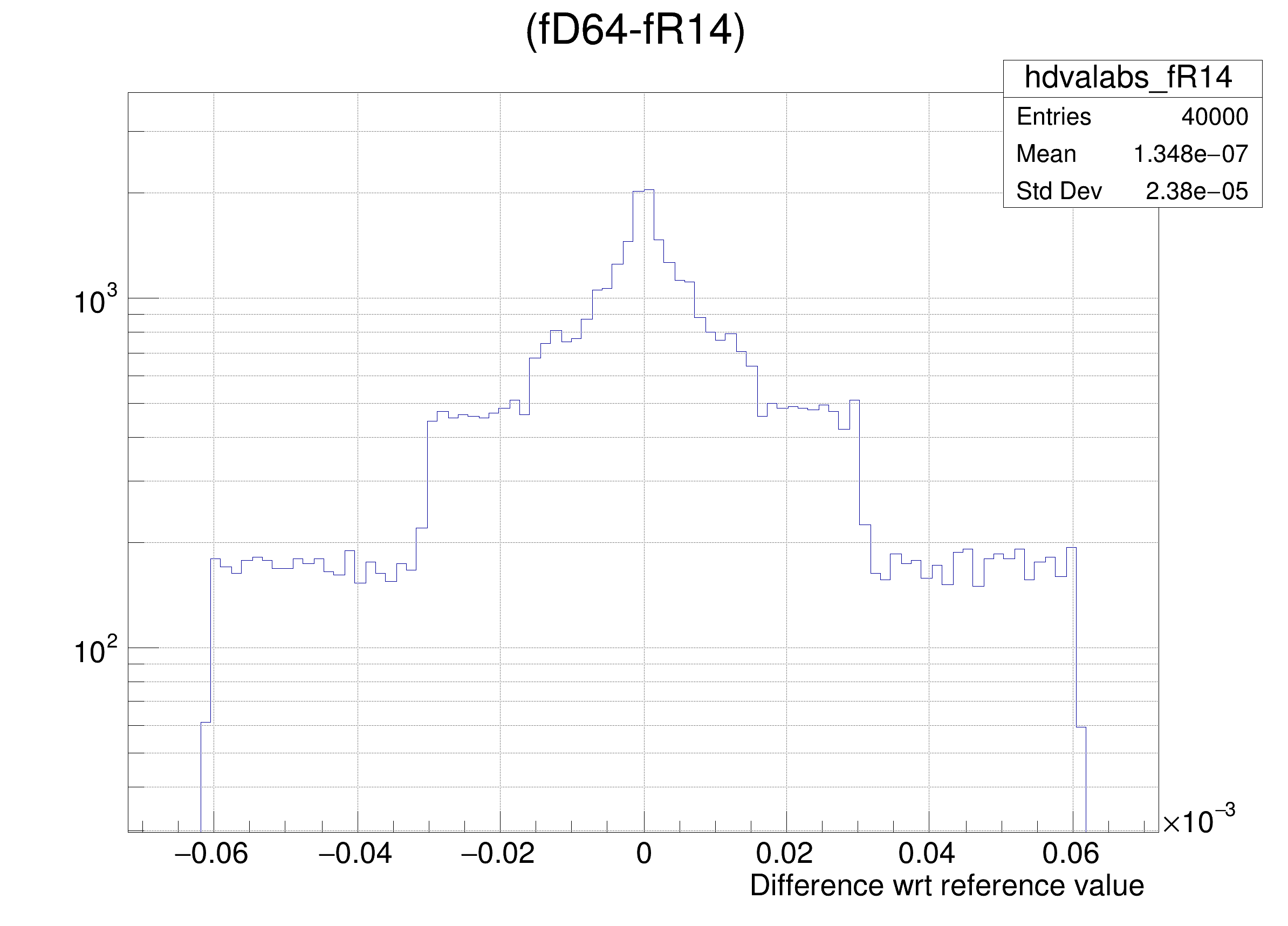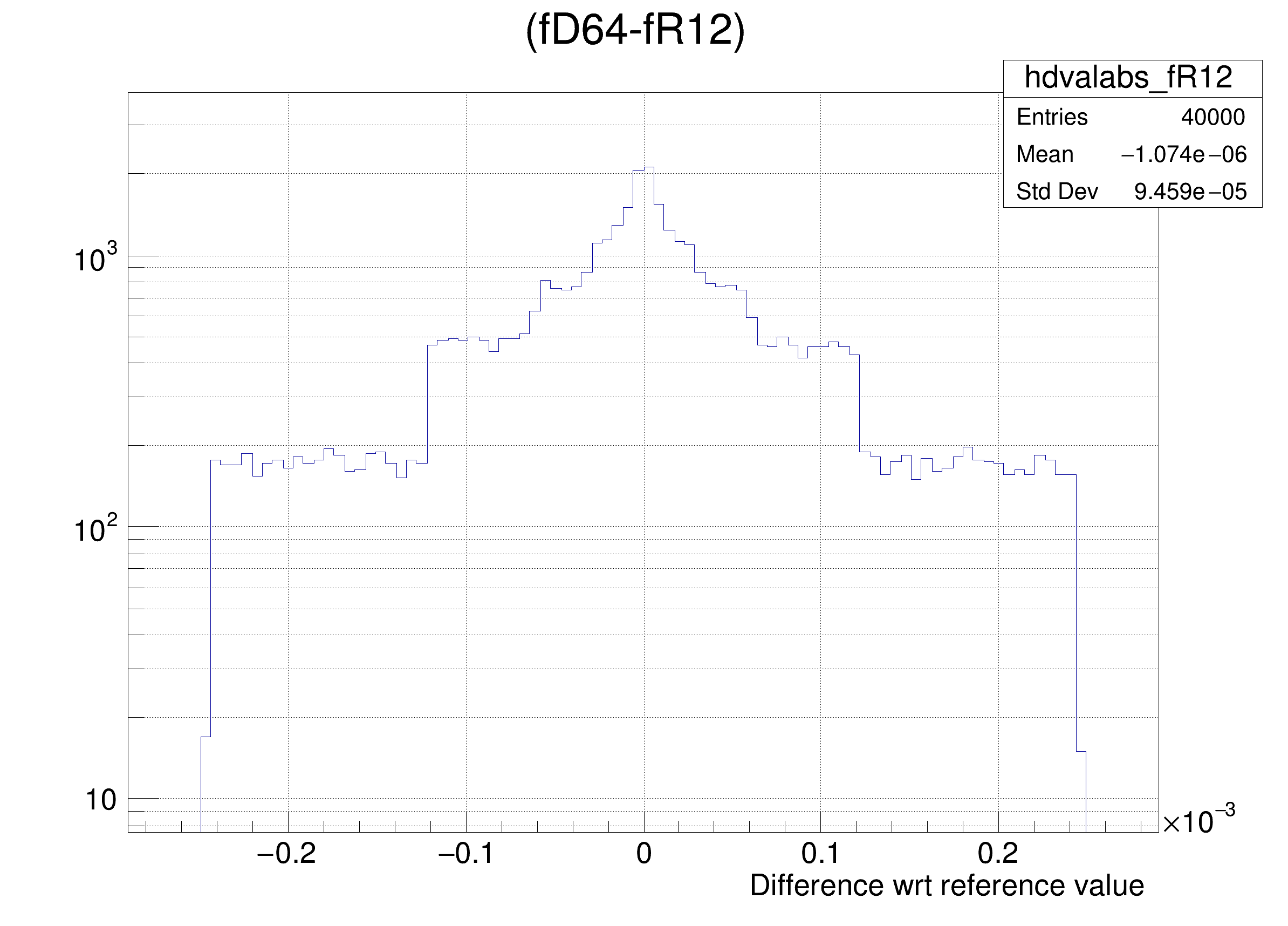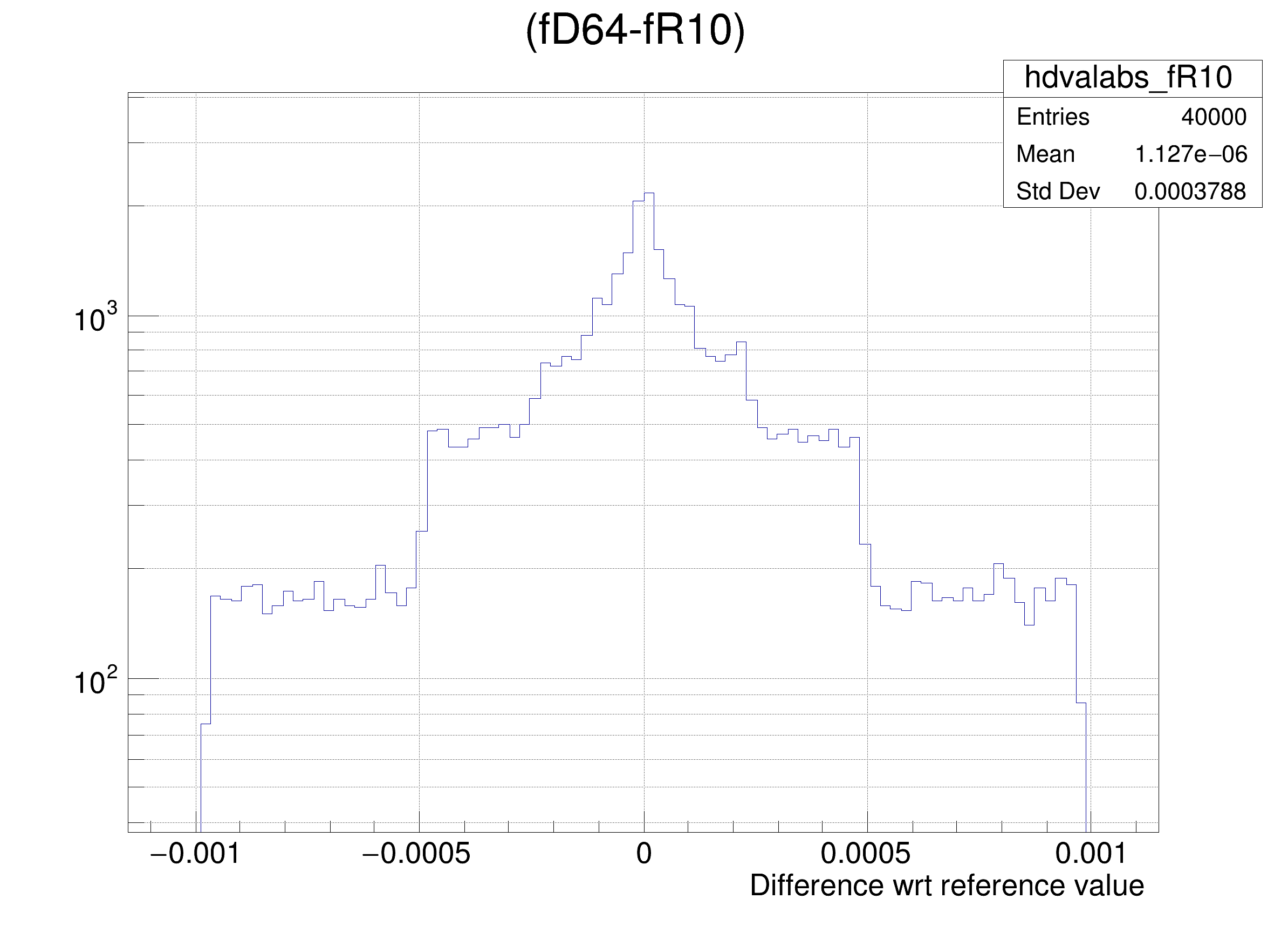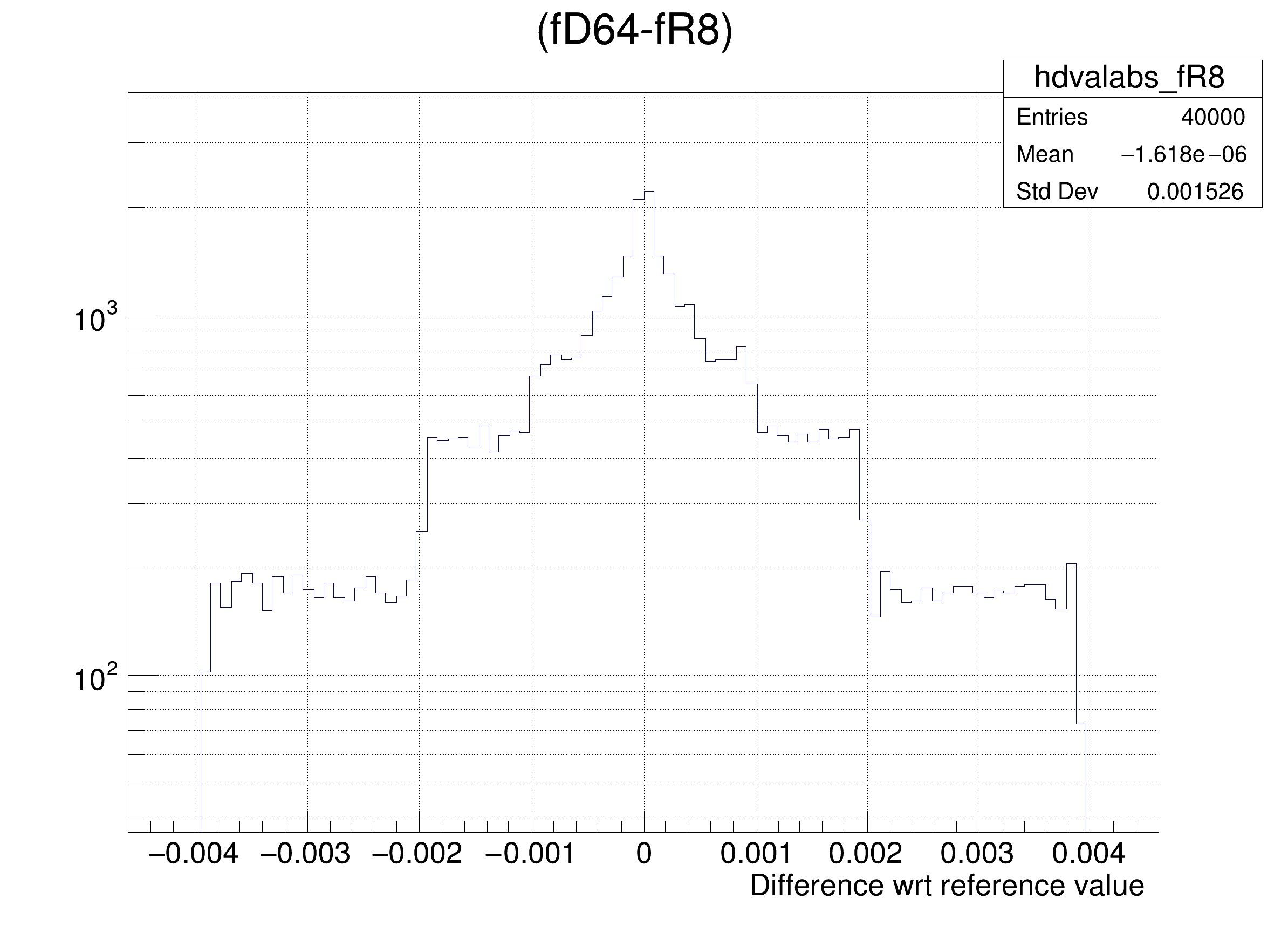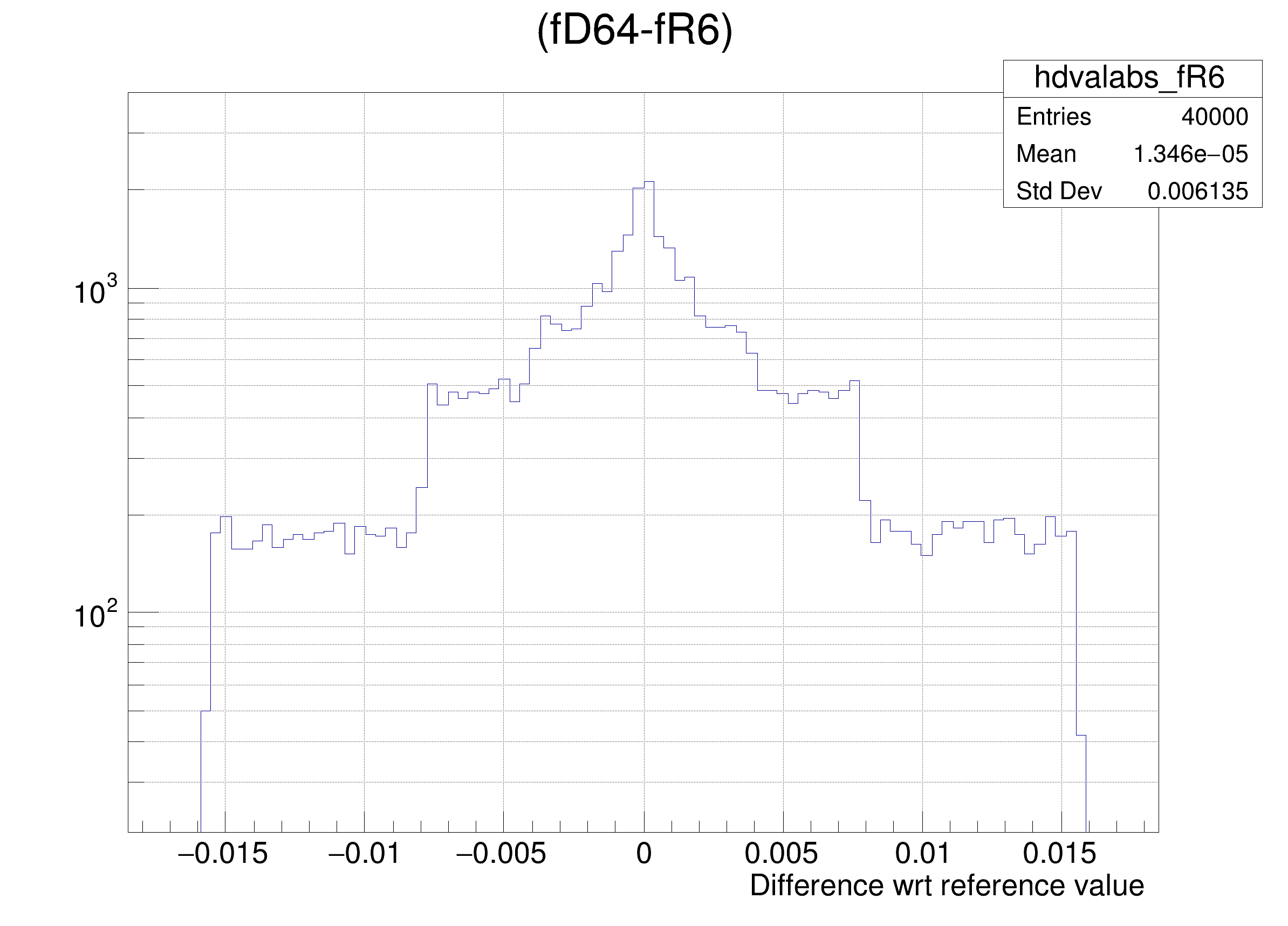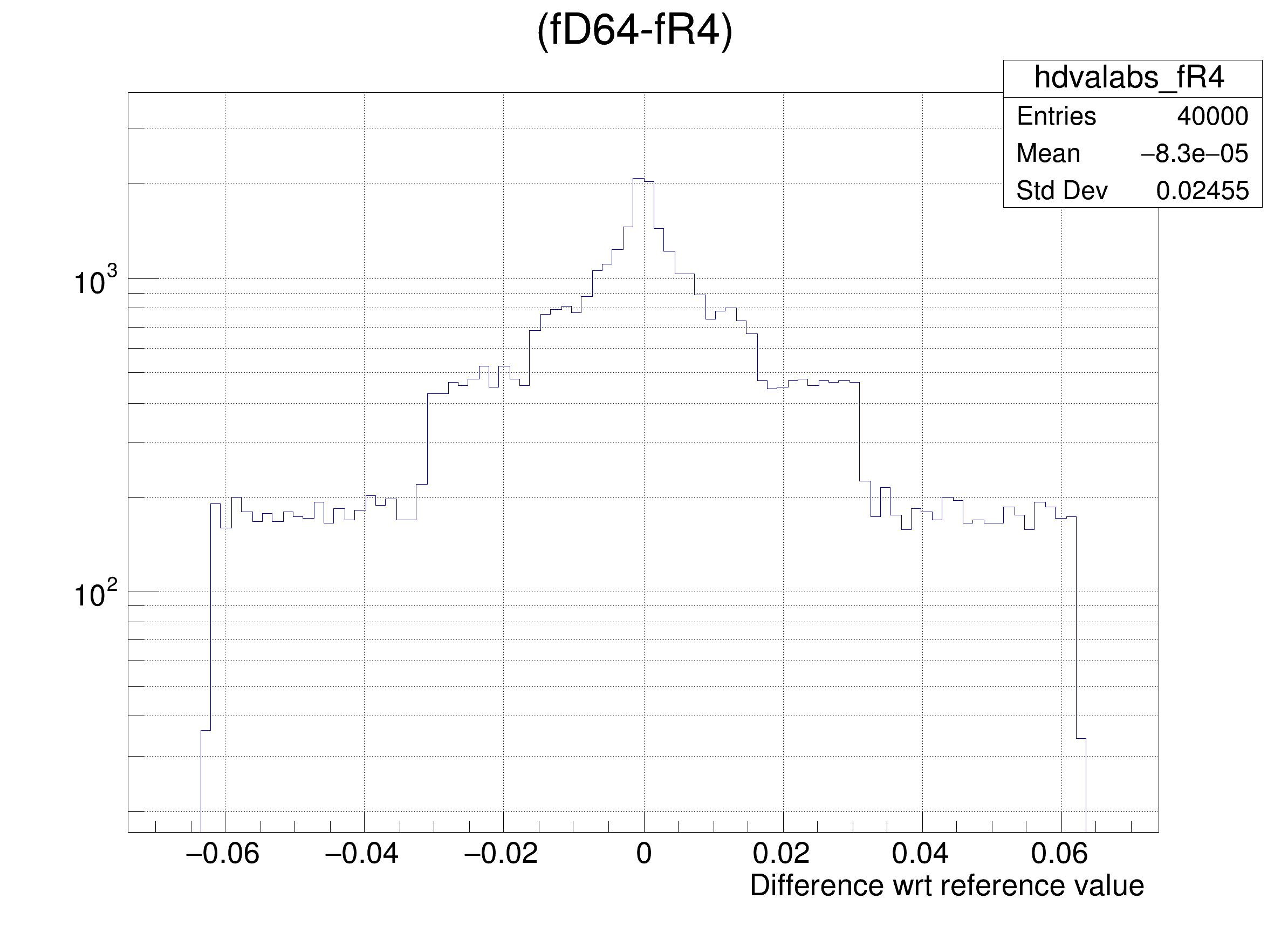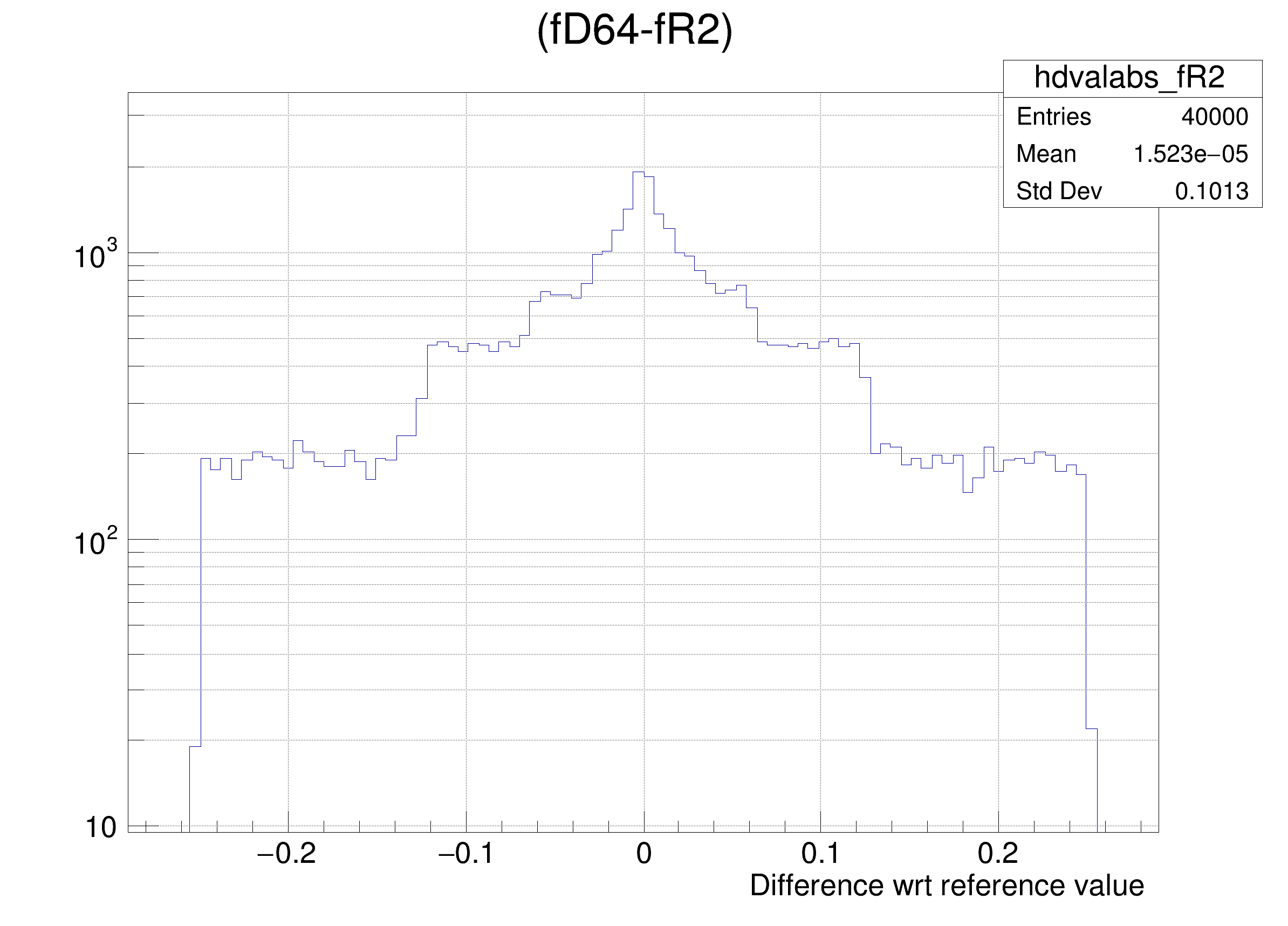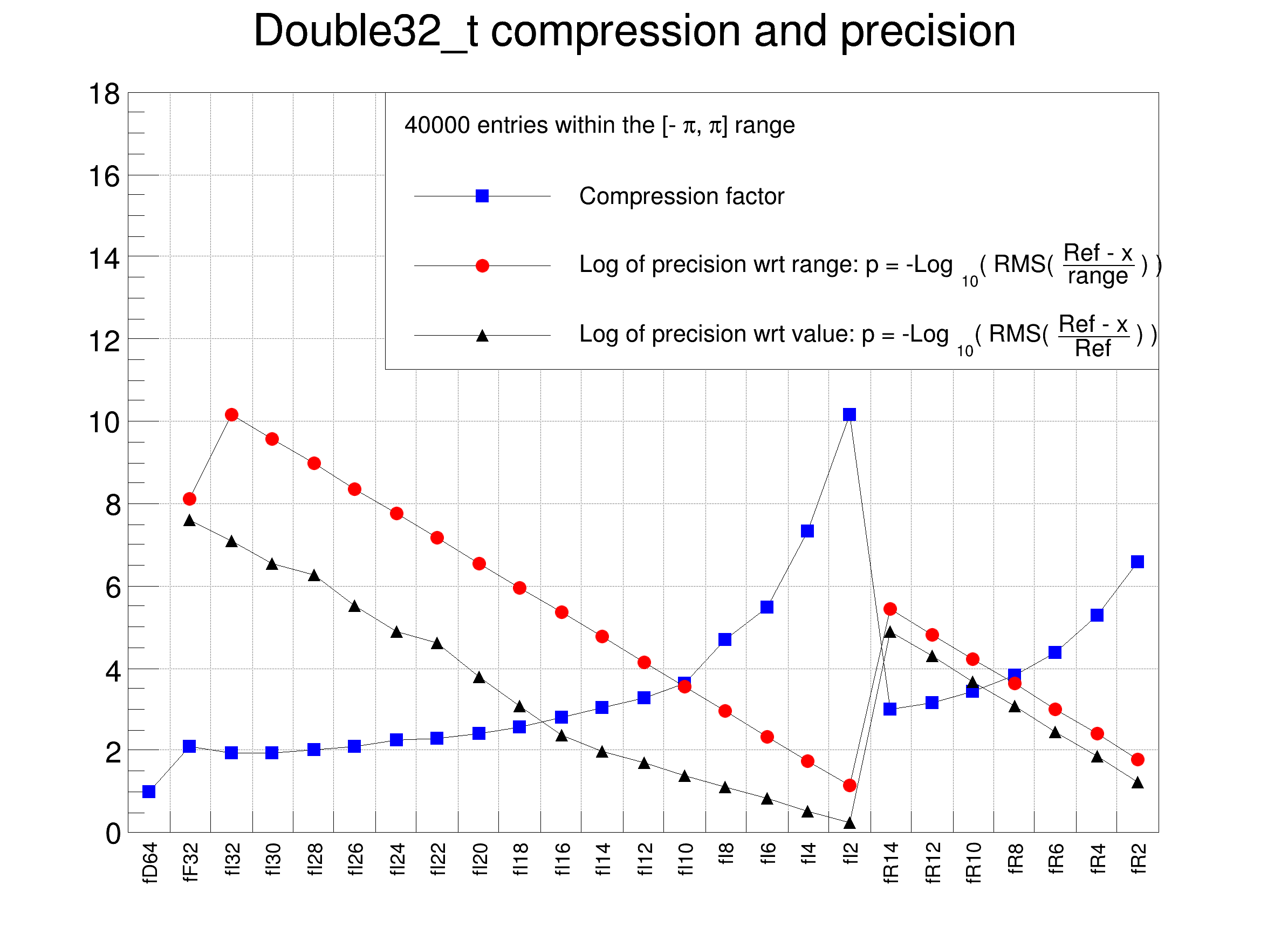#include "ROOT/TSeq.hxx"
#include "TCanvas.h"
#include "TFile.h"
#include "TGraph.h"
#include "TH1.h"
#include "TLegend.h"
#include "TMath.h"
#include "TRandom3.h"
#include "TTree.h"
class DemoDouble32 {
private:
Double_t fD64; // reference member with full double precision
Double32_t fF32; // saved as a 32 bit Float_t
Double32_t fI32; //[-pi,pi] saved as a 32 bit unsigned int
Double32_t fI30; //[-pi,pi,30] saved as a 30 bit unsigned int
Double32_t fI28; //[-pi,pi,28] saved as a 28 bit unsigned int
Double32_t fI26; //[-pi,pi,26] saved as a 26 bit unsigned int
Double32_t fI24; //[-pi,pi,24] saved as a 24 bit unsigned int
Double32_t fI22; //[-pi,pi,22] saved as a 22 bit unsigned int
Double32_t fI20; //[-pi,pi,20] saved as a 20 bit unsigned int
Double32_t fI18; //[-pi,pi,18] saved as a 18 bit unsigned int
Double32_t fI16; //[-pi,pi,16] saved as a 16 bit unsigned int
Double32_t fI14; //[-pi,pi,14] saved as a 14 bit unsigned int
Double32_t fI12; //[-pi,pi,12] saved as a 12 bit unsigned int
Double32_t fI10; //[-pi,pi,10] saved as a 10 bit unsigned int
Double32_t fI8; //[-pi,pi, 8] saved as a 8 bit unsigned int
Double32_t fI6; //[-pi,pi, 6] saved as a 6 bit unsigned int
Double32_t fI4; //[-pi,pi, 4] saved as a 4 bit unsigned int
Double32_t fI2; //[-pi,pi, 2] saved as a 2 bit unsigned int
Double32_t fR14; //[0, 0, 14] saved as a 32 bit float with a 14 bits mantissa
Double32_t fR12; //[0, 0, 12] saved as a 32 bit float with a 12 bits mantissa
Double32_t fR10; //[0, 0, 10] saved as a 32 bit float with a 10 bits mantissa
Double32_t fR8; //[0, 0, 8] saved as a 32 bit float with a 8 bits mantissa
Double32_t fR6; //[0, 0, 6] saved as a 32 bit float with a 6 bits mantissa
Double32_t fR4; //[0, 0, 4] saved as a 32 bit float with a 4 bits mantissa
Double32_t fR2; //[0, 0, 2] saved as a 32 bit float with a 2 bits mantissa
public:
DemoDouble32() = default;
void Set(Double_t ref)
{
fD64 = fF32 = fI32 = fI30 = fI28 = fI26 = fI24 = fI22 = fI20 = fI18 = fI16 = fI14 = fI12 = fI10 = fI8 = fI6 =
fI4 = fI2 = fR14 = fR12 = fR10 = fR8 = fR6 = fR4 = fR2 = ref;
}
};
void double32()
{
const auto nEntries = 40000;
const auto xmax = TMath::Pi();
const auto xmin = -xmax;
// create a Tree with nEntries objects DemoDouble32
TFile::Open("DemoDouble32.root", "recreate");
TTree tree("tree", "DemoDouble32");
DemoDouble32 demoInstance;
auto demoInstanceBranch = tree.Branch("d", "DemoDouble32", &demoInstance, 4000);
for (auto i : ROOT::TSeqI(nEntries)) {
demoInstance.Set(r.Uniform(xmin, xmax));
tree.Fill();
}
tree.Write();
// Now we can proceed with the analysis of the sizes on disk of all branches
// Create the frame histogram and the graphs
auto branches = demoInstanceBranch->GetListOfBranches();
const auto nb = branches->GetEntries();
auto br = static_cast<TBranch *>(branches->At(0));
const Long64_t zip64 = br->GetZipBytes();
auto h = new TH1F("h", "Double32_t compression and precision", nb, 0, nb);
h->SetMaximum(18);
h->SetStats(0);
auto gcx = new TGraph();
gcx->SetName("gcx");
gcx->SetMarkerStyle(kFullSquare);
gcx->SetMarkerColor(kBlue);
auto gdrange = new TGraph();
gdrange->SetName("gdrange");
gdrange->SetMarkerStyle(kFullCircle);
gdrange->SetMarkerColor(kRed);
auto gdval = new TGraph();
gdval->SetName("gdval");
gdval->SetMarkerStyle(kFullTriangleUp);
gdval->SetMarkerColor(kBlack);
// loop on branches to get the precision and compression factors
for (auto i : ROOT::TSeqI(nb)) {
auto br = static_cast<TBranch *>(branches->At(i));
const auto brName = br->GetName();
h->GetXaxis()->SetBinLabel(i + 1, brName);
const auto cx = double(zip64) / br->GetZipBytes();
gcx->SetPoint(i, i + 0.5, cx);
if (i == 0 ) continue;
tree.Draw(Form("(fD64-%s)/(%g)", brName, xmax - xmin), "", "goff");
const auto rmsDrange = TMath::RMS(nEntries, tree.GetV1());
const auto drange = TMath::Max(0., -TMath::Log10(rmsDrange));
gdrange->SetPoint(i-1, i + 0.5, drange);
tree.Draw(Form("(fD64-%s)/fD64", brName), "", "goff");
const auto rmsDVal = TMath::RMS(nEntries, tree.GetV1());
const auto dval = TMath::Max(0., -TMath::Log10(rmsDVal));
gdval->SetPoint(i-1, i + 0.5, dval);
tree.Draw(Form("(fD64-%s) >> hdvalabs_%s", brName, brName), "", "goff");
auto hdval = gDirectory->Get<TH1F>(Form("hdvalabs_%s", brName));
hdval->GetXaxis()->SetTitle("Difference wrt reference value");
auto c = new TCanvas(brName, brName, 800, 600);
c->SetGrid();
c->SetLogy();
hdval->DrawClone();
}
auto c1 = new TCanvas("c1", "c1", 800, 600);
c1->SetGrid();
h->Draw();
h->GetXaxis()->LabelsOption("v");
gcx->Draw("lp");
gdrange->Draw("lp");
gdval->Draw("lp");
// Finally build a legend
auto legend = new TLegend(0.3, 0.6, 0.9, 0.9);
legend->SetHeader(Form("%d entries within the [-#pi, #pi] range", nEntries));
legend->AddEntry(gdrange, "Log of precision wrt range: p = -Log_{10}( RMS( #frac{Ref - x}{range} ) ) ", "lp");
legend->AddEntry(gdval, "Log of precision wrt value: p = -Log_{10}( RMS( #frac{Ref - x}{Ref} ) ) ", "lp");
legend->Draw();
}
ROOT::R::TRInterface & r
Definition: Object.C:4
#define c(i)
Definition: RSha256.hxx:101
#define h(i)
Definition: RSha256.hxx:106
double Double32_t
Definition: RtypesCore.h:56
double Double_t
Definition: RtypesCore.h:55
long long Long64_t
Definition: RtypesCore.h:69
@ kRed
Definition: Rtypes.h:64
@ kBlack
Definition: Rtypes.h:63
@ kBlue
Definition: Rtypes.h:64
@ kFullSquare
Definition: TAttMarker.h:48
@ kFullTriangleUp
Definition: TAttMarker.h:48
@ kFullCircle
Definition: TAttMarker.h:48
#define gDirectory
Definition: TDirectory.h:223
float xmin
Definition: THbookFile.cxx:93
float xmax
Definition: THbookFile.cxx:93
char * Form(const char *fmt,...)
A pseudo container class which is a generator of indices.
Definition: TSeq.hxx:66
A TTree is a list of TBranches.
Definition: TBranch.h:91
Long64_t GetZipBytes(Option_t *option="") const
Return total number of zip bytes in the branch if option ="*" includes all sub-branches of this branc...
Definition: TBranch.cxx:2075
The Canvas class.
Definition: TCanvas.h:31
static TFile * Open(const char *name, Option_t *option="", const char *ftitle="", Int_t compress=ROOT::RCompressionSetting::EDefaults::kUseCompiledDefault, Int_t netopt=0)
Create / open a file.
Definition: TFile.cxx:3923
A Graph is a graphics object made of two arrays X and Y with npoints each.
Definition: TGraph.h:41
1-D histogram with a float per channel (see TH1 documentation)}
Definition: TH1.h:571
TAxis * GetXaxis()
Definition: TH1.h:316
This class displays a legend box (TPaveText) containing several legend entries.
Definition: TLegend.h:23
virtual void SetTitle(const char *title="")
Set the title of the TNamed.
Definition: TNamed.cxx:164
virtual const char * GetName() const
Returns name of object.
Definition: TNamed.h:47
Random number generator class based on M.
Definition: TRandom3.h:27
A TTree represents a columnar dataset.
Definition: TTree.h:72
return c1
Definition: legend1.C:41
Short_t Max(Short_t a, Short_t b)
Definition: TMathBase.h:212
constexpr Double_t Pi()
Definition: TMath.h:38
Double_t RMS(Long64_t n, const T *a, const Double_t *w=0)
Return the Standard Deviation of an array a with length n.
Definition: TMath.h:1157
Double_t Log10(Double_t x)
Definition: TMath.h:754
Definition: tree.py:1

Definition in file double32.C.